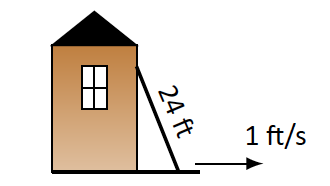# A 24 ft. ladder is leaning against a house while the base is pulled away at a constant rate of 1 ft/s. At what rate is the top of the ladder sliding down the side of the house when the base is:Using Pythagoras theorem, The ladder and house makes up a right triangle: $$x^2+y^2=24^2$$ where x is the horizontal distance the ladder is from the wall and y is the vertical distance the ladder is up the wall. 24 ft. is hypotenuse of the triangle. Firstly, We need to calculate the derivative of this equation that gives us:- $$2x \cfrac { dx }{ dt } + 2y \cfrac { dy }{ dt } =0$$

Here, The Derivative of hypotenuse is 0 as the length of the ladder is not changing.

Solving the problem further gives us $$2y \cfrac { dy }{ dt } = -2x \cfrac { dx }{ dt }$$ or $$y \cfrac { dy }{ dt } = -x \cfrac { dx }{ dt }$$

We know the rate of moving the ladder is 1 ft/s so we can say $$\cfrac { dx }{ dt } = 1$$ Hence, $$y \cfrac { dy }{ dt } = -x$$ or $$\cfrac { dy }{ dt } = \cfrac { -x }{ y }$$

We need to calculate $$\cfrac { dy }{ dt }$$ as it gives us the rate of descent of the ladder. Below cases gives us the value of x with different values. We solve for y and substiture x and y values in the above equation to find the rate of descent. We will solve of y from $$x^2+y^2=24^2$$

dydt <- function(x){
y <- sqrt(24^2 - x^2)
dydtval <- round(-x/y,4)
cat("Rate of descent of ladder is", dydtval, "ft/s.", "\n")
}

# (a) 1 foot from the house?

dydt(1)
## Rate of descent of ladder is -0.0417 ft/s.

# (b) 10 feet from the house?

dydt(10)
## Rate of descent of ladder is -0.4583 ft/s.

# (c) 23 feet from the house?

dydt(23)
## Rate of descent of ladder is -3.3549 ft/s.

# (d) 24 feet from the house?

dydt(24)
## Rate of descent of ladder is -Inf ft/s.